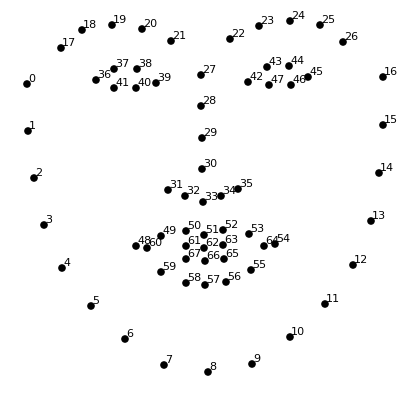# Introduction¶

This notebook contains all the code needed to automatically calculate the Facial-Width-Height ratio based on an image using the face_recognition package.
See: https://github.com/ageitgey/face_recognition

In :
NAME = 'FWHR_calculator'
PYTHON_VERSION = '3.5'
AUTHOR = 'Ties de Kok'


# Preamble¶

## Imports¶

In :
%pylab inline

Populating the interactive namespace from numpy and matplotlib

In :
import math

from matplotlib.pyplot import imshow
from PIL import Image, ImageDraw
import face_recognition

import urllib.request


All the heavy-lifting is done by the face_recognition package, you need to have it installed!
See: https://github.com/ageitgey/face_recognition

# Main Code¶

### Function to load an image¶

This function loads an image from the drive or downloads it from a link if url is set to True.

In :
def load_image(path, url=False):
if not url:
else:
if path[-3:] == 'jpg' or  path[-3:] == 'peg':
urllib.request.urlretrieve(path, 'tmp.jpg')
elif path[-3:] == 'png':
urllib.request.urlretrieve(path, 'tmp.png')
else:
print("Unknown image type")


### Function to get the contour face points¶

This function calculates the coordinates for the corners of the "FWHR" box.
Note 1: it is possible to calculate the top line based on either the bottom of the eyebrows (top = "eyebrow") or the eyelids (top = "eyelid").
Note 2: to counter-act small amounts of rotation it will by default take the average between the height of the two top points.

In :
def get_face_points(points, method='average', top='eyebrow'):
width_left, width_right = points, points

if top == 'eyebrow':
top_left = points
top_right = points

elif top == 'eyelid':
top_left = points
top_right = points

else:
raise ValueError('Invalid top point, use either "eyebrow" or "eyelid"')

bottom_left, bottom_right = points, points

if method == 'left':
coords = (width_left, width_right, top_left, bottom_left)

elif method == 'right':
coords = (width_left, width_right, top_right, bottom_right)

else:
top_average = int((top_left + top_right) / 2)
bottom_average = int((bottom_left + bottom_right) / 2)
coords = (width_left, width_right, top_average, bottom_average)

## Move the line just a little above the top of the eye to the eyelid
if top == 'eyelid':
coords = (coords, coords, coords - 4, coords)

return {'top_left' : (coords, coords),
'bottom_left' : (coords, coords),
'top_right' : (coords, coords),
'bottom_right' : (coords, coords)
}


### Function to check suitability of picture¶

This function checks whether a picture contains a person that is looking straight at the camera.

In :
def good_picture_check(p, debug=False):
## To scale for picture size
width_im = (p - p) / 100

## Difference in height between eyes
eye_y_l = (p + p) / 2.0
eye_y_r = (p + p) / 2.0
eye_dif = (eye_y_r - eye_y_l) / width_im

## Difference top / bottom point nose
nose_dif = (p - p) / width_im

## Space between face-edge to eye, left vs. right
left_space = p - p
right_space = p - p
space_ratio = left_space / right_space

if debug:
print(eye_dif, nose_dif, space_ratio)

## These rules are not perfect, determined by trying a bunch of "bad" pictures
if eye_dif > 5 or nose_dif > 3.5 or space_ratio > 3:
return False
else:
return True


### Function to calculate the FWHR¶

This function calculates the FWHR based on the corners: Width / Height

In :
def FWHR_calc(corners):
width = corners['top_right'] - corners['top_left']
height = corners['bottom_left'] - corners['top_left']
return float(width) / float(height)


### Function to show the FWHR box on the image¶

This function shows the FWHR box on the original image.

In :
def show_box(image, corners):
pil_image = Image.fromarray(image)
w, h = pil_image.size

## Automatically determine width of the line depending on size of picture
line_width = math.ceil(h / 100)

d = ImageDraw.Draw(pil_image)
d.line([corners['bottom_left'], corners['top_left']], width = line_width)
d.line([corners['bottom_left'], corners['bottom_right']], width = line_width)
d.line([corners['top_left'], corners['top_right']], width = line_width)
d.line([corners['top_right'], corners['bottom_right']], width = line_width)

imshow(pil_image)


### Final function that combines the previous functions¶

This function combines all the previous logic into one function.

Arguments:

image_path $\rightarrow$ path or URL to image
url $\rightarrow$ set to True if image_path is a url
show $\rightarrow$ set to False if you only want it to return the FWHR
method $\rightarrow$ determines which eye to use for the top point: left, right, or average
top $\rightarrow$ determines whether to use the eyebrow as top point or eyelid as top point

In :
def get_fwhr(image_path, url=False, show=True, method='average', top='eyelid'):
landmarks = face_recognition.api._raw_face_landmarks(image)
landmarks_as_tuples = [(p.x, p.y) for p in landmarks.parts()]

if good_picture_check(landmarks_as_tuples):
corners = get_face_points(landmarks_as_tuples, method=method, top = top)
fwh_ratio = FWHR_calc(corners)

if show:
print('The Facial-Width-Height ratio is: {}'.format(fwh_ratio))
show_box(image, corners)
else:
return fwh_ratio
else:
if show:
print("Picture is not suitable to calculate fwhr.")
imshow(image)
else:
return None


# Examples¶

## Basic example¶

In :
guy_url = 'http://i.dailymail.co.uk/i/pix/2015/03/30/04/271843AB00000578-0-image-a-86_1427687469357.jpg'
get_fwhr(guy_url, url=True)

The Facial-Width-Height ratio is: 1.9367088607594938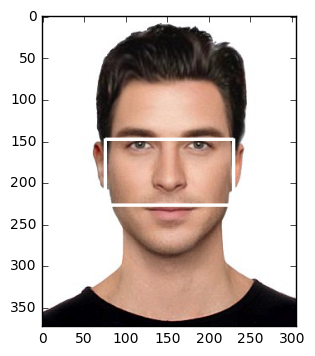## Difference eyelid vs. eyebrow¶

In :
obama_url = 'http://mienshiang.com/wp-content/uploads/images-29.jpg'
get_fwhr(obama_url, url=True, top = 'eyelid')

The Facial-Width-Height ratio is: 1.9285714285714286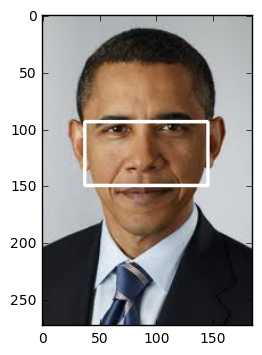In :
get_fwhr(obama_url, url=True, top = 'eyebrow')

The Facial-Width-Height ratio is: 1.8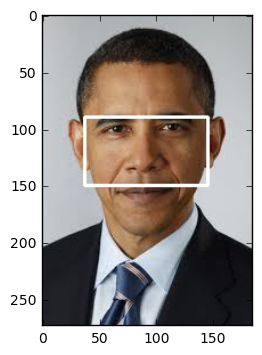## FWHR only¶

In :
get_fwhr(obama_url, url=True, top = 'eyebrow', show=False)

Out:
1.8

In :
bad_img_url_1 = 'https://s-media-cache-ak0.pinimg.com/originals/0d/02/d2/0d02d28791fab2a6f056648dc174033c.jpg'

Picture is not suitable to calculate fwhr.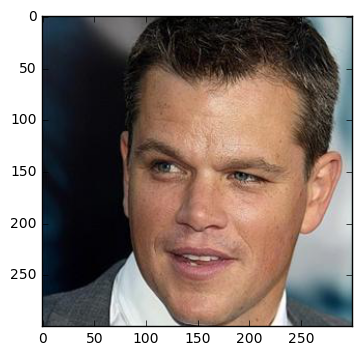In :
bad_img_url_2 = 'https://s-media-cache-ak0.pinimg.com/originals/35/7f/f6/357ff6776c8ec6f565d06e05f902f88b.jpg'

Picture is not suitable to calculate fwhr.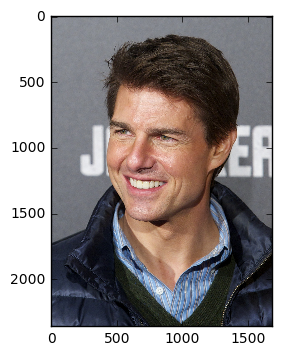# Explanation¶

The data points used in the functions above use this picture as reference:

In :
from IPython.display import Image as ImageShow
ImageShow(url = 'https://cdn-images-1.medium.com/max/1600/1*AbEg31EgkbXSQehuNJBlWg.png')

Out: